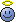# Cauchy-Euler Diff. Eqn

• I
Gold Member
Cauchy-Euler is a type of diff equation which is described by

$$a_0x^2(\frac {d^2y} {dx^2})+a_1x(\frac {dy} {dx})+a_2y=F(x)$$

The transformation of ##x=e^t## can solve the equation.

Now, in here I didnt understand how to transform ##\frac {dy} {dx}## to ##\frac {dy} {dt}##.

it goes like this ##\frac {dy} {dx}=\frac {dy} {dt} \frac {1} {x}## and then I am stuck I should take another derivative but I couldnt do it somehow.

Last edited:

Orodruin
Staff Emeritus
Homework Helper
Gold Member
Let ##dy/dt = f## and apply the product rule for derivatives when differentiating ##f/x## wrt x.

Gold Member
Let ##dy/dt = f## and apply the product rule for derivatives when differentiating ##f/x## wrt x.
Okay hmm

##\frac {dy} {dx}=\frac {f} {x}##
##\frac {d^2y} {dx^2}=\frac {df} {dx} \frac {1} {x}-\frac {f} {x^2}##

now I understand until here, but I didnt understand this term ##\frac {df} {dx}=\frac {d^2y} {dt^2}\frac {1} {x}## which its done in the book.

Orodruin
Staff Emeritus
I understand it now, thanks